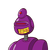# 7. If the sum of the zeroes of the polynomial P(x)=2x³-3 kx²+ 4x -5 is 6, then Find the valueof ‘k’​

7. If the sum of the zeroes of the polynomial P(x)=2x³-3 kx²+ 4x -5 is 6, then Find the value
of ‘k’​

### 1 thought on “7. If the sum of the zeroes of the polynomial P(x)=2x³-3 kx²+ 4x -5 is 6, then Find the value<br />of ‘k’​”

1.k=4

explanation :

sum of zeroes = -b/a( b= coefficient of x^2, a=coefficent of x^3)

given sum of zeroes=6

6=-(-3k)/2

6×2=3k

12=3k

12/3=k

4=k